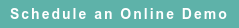# Blog

### Posts by Topic

To grasp the legalese of my initial encounters with the 1968 US transfer pricing regulations (under section 482 published in the Federal Register (33 FR 5848), April 16, 1968), I translated the three specified transfer pricing methods (CUP, resale price and cost plus) into algebra and found a multiplier formula tying them together.

I created a two equation system including an accounting equation and a stochastic equation, and obtained the reduced-form equation to estimate the price (CUP) or the selected gross profit indicator. Using the same multiplier procedure, I developed the CPM/TNMM in 1989.

#### Reduced-Form Markup Pricing Equation

Thus, from a single reduced-form equation, the CUP, resale price, cost-plus methods, and the novel CPM (comparable profits method) can be derived:

(1)     p = (c + z), which means that unit price = unit cost plus profit.

(2)     z = µ p, which is a stochastic profit margin equation and µ is the profit margin.

(3)     p = λ c, which is the reduced-form markup price (CUP) equation.

The reduced-form slope coefficient λ = 1 / (1 − µ) is the profit markup, and the derived (indirect least squares, ILS) coefficient µ = (λ – 1) / λ is the profit margin. Unit price, cost, and profit are scalars or row vectors. The regression parameters are scalars.

If a CUP cannot be found, equation (3) is multiplied by q, the quantity sold, and (like in classical economics) revenue becomes proportional to total cost:

(4)     p q = λ c q, or

(5)     R = λ C

The quantity variable is a scalar or column vector. The profit markup λ multiplier remains the same after aggregation over many products. This single reduced-form equation shows that the 1968 regulations contained one pricing equation expressed in disaggregated (equation (3)) or aggregated (equation (5)) form. The notion of three transfer pricing methods is innocent because the CUP and profit margin equations are nested inside the (general) price markup equation (5).

The difference between gross profit and operating profit methods is the concept of cost employed in the analysis and the resulting concept of profit. The reduced-form equation is the same, only the cost variable is measured as COGS alone or COGS plus XSGA (operating expenses).

If cost = COGS, the analysis pertains to the unreliable gross profit paradigm of the 1968 regulations. If cost = (COGS + XSGA), the analysis belongs to the reliable operating profit paradigm of the 1994 regulations. The comparable profits method (CPM/TNMM), quorum pars magna fui, was incorporated in the 1994 regulations and then morphed into the TNMM of the 1995 OECD guidelines.

Remembrance of Things Past

Christine Halphen, Special Counsel at the IRS Office of Chief Counsel, described the incipient CPM on two memoranda (in which I was copied) written on August 20, 1990 and March 28, 1991. In part, she wrote: “In a memorandum dated August 20, 1990, our office [Chief Counsel] had advised the Manhattan Examination District that we would support the use of statistical data to determine pricing adjustments under two conditions. First, we indicated that, in order to stand a chance in litigation, the statistical sample must consist of companies that bear sufficient similarity to the taxpayer. Also, through the issuance of IDRs [Information Document Requests] at the beginning of each audit, the IRS must make an effort to ascertain whether CUPs exist or whether the taxpayer has competitors [comparables] in the U.S. that might be used as independent resellers in order to apply the resale price method.”

“Ednaldo Silva, an economist with the Manhattan District, presented a proposal for building an industry specific statistical database … The Manhattan District has already started to apply a statistical method [comparable profits interval] to determine pricing adjustments in a number of small cases and is reporting a number of settlements and agreements in exam. It is obvious that this approach is simplifying our enforcement effort as well as encouraging compliance and will continue to do so until such time as the courts decide, if they ever do, that the IRS cannot use statistical methods to make pricing adjustments. To enhance our litigation stance, it might be useful to give recognition to the method in the regulations.”

In 1991, I became the first economist in the IRS Office of Chief Counsel and was transferred from the IRS Manhattan District to Washington DC to assist counsel incorporate the comparable profits method in the section 482 regulations. I became also the first economist in the nascent APA program, and I used the APA as a nursery school to further test the CPM.

I learned the multiplier economic modelling from Oskar Lange, Introduction to Economic Cybernetics, Pergamon Press, 1970 and later (using Keynes-Samuelson economic applications) from Roy Allen, Mathematical Economics (2nd edition), Macmillan, 1959, Chapter 9 (Closed-loop control systems). Allen was referenced by Lange. Multiplier theory is diffuse in economics, including the scalar form of the Kahn-Keynes and Kalecki investment multipliers and the Leontief matrix multiplier. On my blogs, I continue to interpret transfer pricing in terms of multiplier theory. E.g., please see:https://blog.royaltystat.com/creating-defensible-transfer-pricing-reports

Published on Jan 6, 2021 1:04:37 PM

Ednaldo Silva (Ph.D.) is founder and managing director of RoyaltyStat. He helped draft the US transfer pricing regulations and developed the comparable profits method called TNNM by the OECD. He can be contacted at: esilva@royaltystat.com

RoyaltyStat provides premier online databases of royalty rates extracted from unredacted license agreements
and normalized company financials (income statement, balance sheet, cash flow). We provide high-quality data, built-in analytical tools, customer training and attentive technical support.